﻿

### Products of groups and local nearrings

#### Abstract

Groups which can be written as a product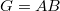$G=AB$ of two of its subgroups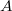$A$ and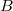$B$ have been studied by many authors. A particular role in such investigations play groups of the form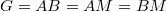$G=AB=AM=BM$ where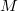$M$ is a normal subgroup of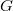$G$ and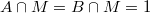$A\cap M=B\cap M=1$. It turns out that there is a close connection between groups of this form and some nearrings, especially so called local nearrings, so that many problems concerning such nearrings can be reduced to questions about these groups. In the following we will discuss different aspects of this connection and, in addition, consider in detail certain structural questions arised in the case of almost cyclic subgroups$A$ an$B$.

DOI Code: 10.1285/i15900932v28n2supplp177

Keywords:
factorized group; derivation; local nearring; multiplicative group

Full Text: PDF